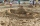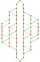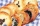Examples for 8th grade - page 113

1. Price inflationThis year the average wage grew by 4.5%. Prices rose by 2%. How much did the real wage increase in the given year?
2. Sand castleTim and Tom built a sand castle and embellished it with a flag. Half the pole with the flag plunged into the castle. The highest point of the pole was 80 cm above the ground, its lowest point 20 cm above the ground. How high was the sand castle?
3. Mba studiumAt MBA school, fourth-year students can choose from three optional subjects: a) mathematical methods, b) social interaction, c) management Each student studies one of these subjects. The mathematical methods studied 28 students, the social interaction 27.
4. ThomasThomas lives 400 meters away from Samko, Robo from Thomas also 400 m and Samko from Robo 500. Anton lives 300 meters away from Robo further as Samko. How far away lives Anton from Rob?
5. DivideDivide area of rectangles with dimensions 32m and 10m by the ratio 7: 9. What area corresponds to a smaller section?
6. Diofant equation250x + 120y = 5640
7. Two unknown numbersOne number is 2 less than a second number. Twice the second number is 14 less than 5 times the first. Find the two numbers.
8. Midpoint 4If the midpoint of a segment is (6,3) and the other end point is (8,-4) what are thw coordinate of the other end?
9. Combinations 7A multi-course meal has 3 choices. 3 appetizers, 4 main courses, and 3 desserts. How many different combinations to choose from?
10. Linear systemSolve a set of two equations of two unknowns: 1.5x+1.2y=0.6 0.8x-0.2y=2
11. 5 people5 people have \$122000 and 1 person has \$539000 How much should each person (equally) pay?Montealegre family has a catering business. In one birthday party, they charged their costumer 11,767.50 for a party of 90 persons and a service charge of 2,500.50. How much was the average cost per person? Please show your solution.
13. Arble bagA marble bag sold by Rachel's Marble Company contains 5 orange marbles for every 6 green marbles. If a bag has 35 orange marbles, how many green marbles does it contain?
14. Value 4If 5/18=425 what is the value 13/18?
15. Reservoir 3How many cubic feet of water is stored in the reservoir that has a length of 200 feet, a width of 100 feet, an overflow depth of 32 feet, and a current water level of 24 feet?
16. Pages countingThere are pages numbered from 2 to 104 in the book. How many digits have to be printed to number the pages?
17. Jane plantsJane plants flowers in the garden. If she planted 12 every hour instead of 9 flowers, she would finish with the job an hour earlier. How many flowers does she plant?
18. Pile of sandA large pile of sand has been dumped into a conical pile in a warehouse. The slant height of the pile is 20 feet. The diameter of the base of the sand pile is 31 feet. Find the volume of the pile of sand.
19. Odd/even numberPick any number. If that number is even, divide it by 2. If it's odd, multiply it by 3 and add 1. Now repeat the process with your new number. If you keep going, you'll eventually end up at 1. Every time. Prove. ..
20. SimplifySimplify expression - which expression is equivalent to: 3(m + 2) − 4(2m − 9)

Do you have an interesting mathematical example that you can't solve it? Enter it, and we can try to solve it.

To this e-mail address, we will reply solution; solved examples are also published here. Please enter e-mail correctly and check whether you don't have a full mailbox.